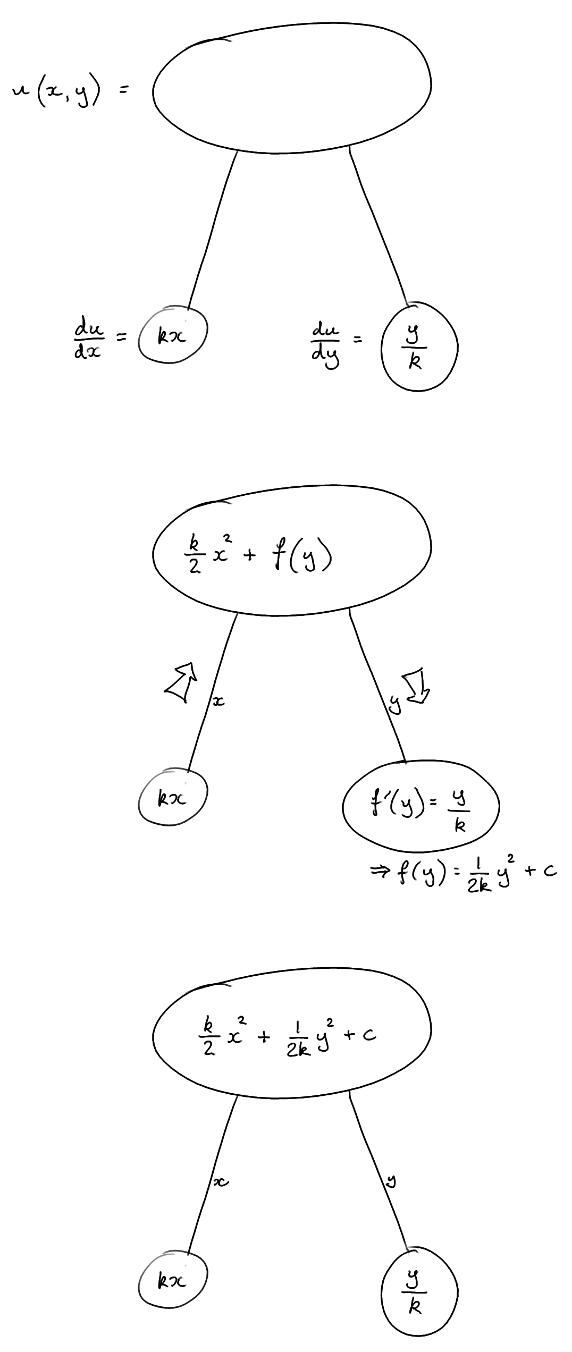# Thread: partial differential equation

1. ##partial differential equation

Let u = u(x,y) be the complete integral of the PDE

$\displaystyle \frac {\partial u} {\partial x} . \frac {\partial u} {\partial y} = xy$ passing through the points
(0,0,1) and (0,1,1/2) in the x-y-u space.

Compute the value of u(x,y) evaluated at (-1,1) is _____

I'm completely lost with respect to this problem.. 'd be great if someone can show me the right direction.

Thanks,

2. ## Re: partial differential equation

\displaystyle \displaystyle \begin{align*} \\ \frac {\partial u} {\partial x} . \frac {\partial u} {\partial y}\ &=\ xy &=\ (kx) (\frac{y}{k})\ & \Leftarrow\ \LARGE{[}\ \frac {\partial u} {\partial x} = kx \And \frac {\partial u} {\partial y} = \frac{y}{k}\ \Huge{]} \end{align*}

Spoiler:

Just in case a picture helps...Spoiler:

\displaystyle \displaystyle \begin{align*} \text{E.g.,}\ \ u(0,0)\ =\ 1\ =\ \frac{k}{2}(0)^2 + \frac{1}{2k}(0)^2 + c\ \Rightarrow\ c = 1 \\ \\ \text{And, e.g.,}\ \ u(0,1)\ =\ \frac{1}{2}\ =\ \frac{k}{2}(0)^2 + \frac{1}{2k}(1)^2 + 1\ \Rightarrow\ \text{etc.} \end{align*}

_________________________________________

Don't integrate - balloontegrate!

Balloon Calculus; standard integrals, derivatives and methods

Balloon Calculus Drawing with LaTeX and Asymptote!

3. ## Re: partial differential equation

Tom, thanks for the reply! that definitely showed a lot of light on how to get started !!! Thanks again, TOM@ballooncalculus... The image was thoughtfully designed...#### Search Tags

differential, equation, partial, pde